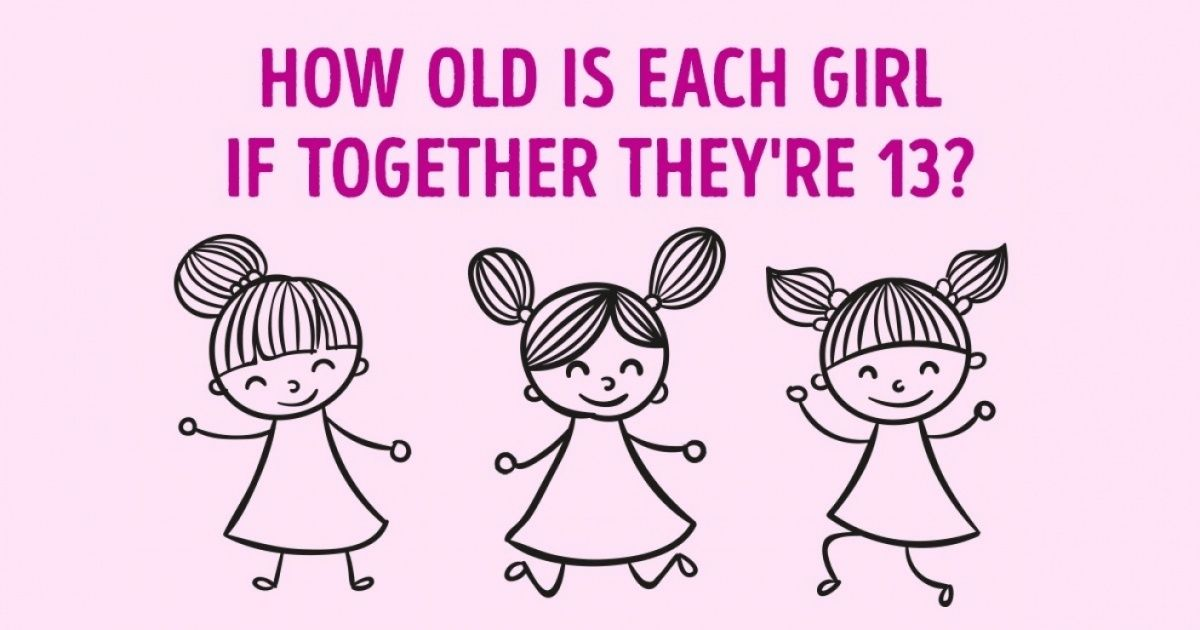# Can You Solve Brainteasers That Not Even Computers Understand?

Would you be able to solve these brainteasers if they were given to you on a computing course?

### Girls ageWhat are the ages of these three sisters if:

When you multiply their ages together, you get 36; when you add their ages together, you get 13. The oldest sister is blonde.
So, did you manage to figure it out?Girls age:

If multiplying their ages yields 36, then their ages must fall into one of the eight categories below:

36 = 2 3 6, and when these ages are added together, we get 11.

36 = 2 2 9, and when these ages are added together, we get 13.

36 = 4 9 1, and when these ages are added together, we get 14.

36 = 4 3 3, and when these ages are added together, we get 10.

36 = 18 2 1, and when these ages are added together, we get 21.

36 = 12 3 1, and when these ages are added together, we get 16.

36 = 6 6 1, and when these ages are added together, we get 13.

36 = 36 1 1, and when these ages are added together, we get 38.

Since 2 + 2 + 9 Equals 13, as does 6 + 6 + 1, we’re left with two options. This is when the seemingly insignificant remark that “the oldest sister is blonde” comes in handy. This phrase denotes the existence of an elder sister who is not a twin. As a result, only the first equation is valid. As a result, the eldest sister is 9 years old, while the younger sisters are both 2.

Spiderman:

The third image from the right in the second row.